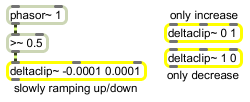# deltaclip~

Limit changes in signal amplitude

## Description

deltaclip~ limits the change between samples in an incoming signal. It is similar to the clip~ object, but it limits amplitude changes with respect to slope rather than amplitude.

## Arguments

Name Type Opt Description
minimum and maximum-slope-values float opt Initial minimum and maximum slope values for the rate of change of the output signal. If no argument is supplied, the minimum and maximum limits are both initially set to 0. If a signal is connected to the middle or right inlet, the corresponding argument is ignored.

## Messages

 int minimum and maximum-slope-value [int] In middle inlet: Minimum slope for the rate of change of the output signal. The minimum slope is typically negative. In right inlet: Maximum slope for the rate of change of the output signal. The maximum slope is typically positive. float minimum and maximum-slope-value [float] In middle inlet: Minimum slope for the rate of change of the output signal. The minimum slope is typically negative. In right inlet: Maximum slope for the rate of change of the output signal. The maximum slope is typically positive. (inlet1) minimum-slope-value [float] Performs the same function as a float in the middle inlet. (inlet2) maximum-slope-value [float] Performs the same function as a float in the right inlet. reset Resets the minimum and maximum slope values to the default value of 0. signal In left inlet: Any signal.

## Output

signal: The input signal is sent out, with its change limited by the minimum and maximum slope values.

## ExamplesLimit a signal's rate of change